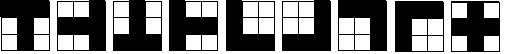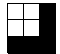You may also likeNumber Detective

Follow the clues to find the mystery number.Score

There are exactly 3 ways to add 4 odd numbers to get 10. Find all the ways of adding 8 odd numbers to get 20. To be sure of getting all the solutions you will need to be systematic. What about a total of 15 with 6 odd numbers?Great Granddad is very proud of his telegram from the Queen congratulating him on his hundredth birthday and he has friends who are even older than he is... When was he born?

Prime Magic

Age 7 to 16 Challenge Level:

Solutions to aspects of this problem have been submitted by Coope, Wern and Adam. I have used their solutions as the basis of what follows. Many thanks to each of you for your efforts and well done.

Since each row must add up to a prime number and the lowest number possible on any row, column or diagonal is 1+2+3 = 6, you can use the restriction that all totals of rows and columns must be odd as all primes greater than 2 are odd.

Using this information let us first consider the case for making horizontal and vertical sets prime. The restriction of 5 odd numbers means that to satisfy the conditions given above the following formation must be present:
1. One row contains 3 odd numbers, and the remaining 2 contain one odd number each.
2. One column contains 3 odd numbers, and the remaining 2 contain one odd number each.

There are 9 possible arrangements for the odd numbers. 4 are rotations of a T shape, 4 are rotations of an L shape and there's one that's a cross shape.These are the only configurations of odd numbers that will allow for the horizontal and vertical sets to possibly sum to a prime. However, out of all of these, the only possible arrangement that could satisfy the diagonal property is the 'cross' shape, as all the others have an even number of odd numbers on at least one diagonal.

It's important to note that swapping a pair of rows or a pair of columns doesn't affect the row and column sums (you should check this is true). However, these swaps do affect the diagonal sums. To make it easier to be systematic, let's just consider squares of the following form, with all the odd numbers in the third row or the third column (as all other squares can be changed into this form by row and column swaps):We know we can swap rows and columns to get our square into this form without affecting the row sums and the column sums. Let's not worry about the diagonal sums for the moment.

It turns out there are 16 possible squares of this form, which are listed in this table according to which odd number is in the bottom right corner. Intriguingly, there are no squares with 5 in the bottom right corner.

 2 8 3 4 6 9 7 5 1 2 8 3 6 4 9 5 7 1 2 8 9 6 4 3 5 7 1 2 8 9 4 6 3 7 5 1 Have 1 in bottom right corner and can be transformed to put 1 in centre and even nos. at the corners (see below for an example) 2 8 3 6 4 9 5 1 7 2 8 3 4 6 9 1 5 7 2 8 9 6 4 3 5 1 7 2 8 9 4 6 3 1 5 7 Have 7 in bottom right corner and can be transformed to put 7 in centre and evens nos. at the corners 2 8 1 6 4 7 9 5 3 2 8 1 4 6 7 5 9 3 2 8 7 6 4 1 9 5 3 2 8 7 4 6 1 5 9 3 Have 3 in bottom right corner and can be transformed to put 3 in centre and evens nos. at the corners 2 8 1 6 4 7 3 5 9 2 8 1 4 6 7 5 3 9 2 8 7 6 4 1 3 5 9 2 8 7 4 6 1 5 3 9 Have 9 in bottom right corner and can be transformed to put 9 in centre and evens nos. at the corners

Now let's see if any of these also satisfy the property that their diagonals also sum to a prime. We know the only possible arrangement that will satisfy this is the 'cross', with all the even numbers at the corners. Let's try the first one, in this case ending up with 1 in the centre:

 2 8 3 4 6 9 7 5 1 swapping second and  third column gives 2 3 8 4 9 6 7 1 5 swapping second and third row gives: 2 3 8 7 1 5 4 9 6

The final square is of the 'cross' form, but its diagonals sum to 9 and 13, so it doesn't satisfy the diagonal property as well as the row and the column property.

If this process is repeated for all the others, it turns out none of them have the diagonal property as well as the row and the column property, so it's not possible to have all the rows, columns and diagonals summing to a prime using the rules we have.

The following table shows all the above solutions in the 'cross' arrangement, and it's easily verified that in each one at least one of each of the diagonals doesn't add to a prime.

 238 715 496 238 517 694 298 517 634 298 715 436 238 571 694 238 175 496 298 571 634 298 175 436 218 935 674 218 539 476 278 935 614 278 539 416 218 395 674 218 593 476 278 395 614 278 593 416